University of Bialystok - Central Authentication System You are not logged in | log in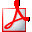Print syllabus

# Probability Calculus I

## General data

 Course ID: 0600-MS1-3RP1#a Erasmus code / ISCED: 11.103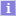/ (0541) MathematicsCourse title: Probability Calculus I Name in Polish: Probability Calculus I Department: Faculty of Mathematics and Informatics Course groups: (in Polish) 3L stac. I st. studia matematyki - przedmioty obowiązkowe ECTS credit allocation (and other scores): 4.00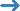view allocation of credits Language: English Type of course: obligatory courses Prerequisites: Combinatorics 0600-MS1-1KOM#aMathematical Analysis III 0600-MS1-2AM3#a Short description: Course objectives: By the end of the course the student should understand fundamental concept of probability and be able to use probabilistic methods in practice. Full description: Course profile: academic Form of study: stationary Course type: obligatory Academic discipline: Mathematics, field of study in the arts and science: mathematics Year: 3, semester: 5 Prerequisities: Mathematical Analysis III, Combinatorics lecture 30 h. exercise class 30 h. Verification methods: lectures, exercises, consultations, studying literature, home works, discussions in groups. ECTS credits: 4 Balance of student workload: attending lectures15x2h = 30h attending exercise classes 7x4h + 2h(preliminary instructions) = 30h preparation for classes 7x3h = 21h completing notes after exercises and lectures 7x2h = 14h consultations 5x2h = 10h the final examination: preparation.and take 15h + 4h = 19h Quantitative description Direct interaction with the teacher: 74 h., 2 ECTS Practical exercises: 75 h., 3 ECTS Learning outcomes: Learning outcomes: Student has basic knowledge about problems of classical probability such that the low of large number and limit theorems.K_W04, K_W05 Student knows the concept of probability and its basic properties.K_W05, K_W12 Student knows basic probability schemes, including Bernoulli's scheme.K_W03, K_W05 Student is able to give examples of discrete and continuous probability distributions and discuss selected random experiments and mathematical models in which these distributions appear.K_U31, K_U33, K_W02, K_W05 Student is able to determine the basic parameters of the distribution of the random variable.K_U31, K_U33, K_W05 Student is able to build a probabilistic model for a given random event and choose method to calculate probability.K_U30, K_U31, K_U32, K_U33 Student is able to apply basic schemes of probability, including the formula for the total probability and the Bayes' formula.K_U30, K_U31, K_U32, K_U33, K_W05 Student is able to describe discrete random phenomena in the surrounding world using probabilistic language and concepts.K_W03, K_U30, K_W12 Student knows the limitations of his knowledge and understands the need of further learning in field of probability.K_K01 Assessment methods and assessment criteria: The overall form of credit for the course: final exam

## Classes in period "Academic year 2018/2019" (in progress)

 Time span: 2018-10-01 - 2019-06-30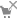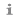Choosen plan division: this week course termsee course schedule Type of class: Class, 30 hoursmore information Lecture, 30 hoursmore information Coordinators: Jarosław Kotowicz Group instructors: (unknown) Students list: (inaccessible to you) Examination: Examination
Course descriptions are protected by copyright.
Copyright by University of Bialystok.